### Thermodynamics Relations - Proof 10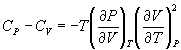From the realtionships between specific heat and entropy, as given in Equn.3.3 and Equn.4.4,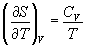→ (10.1)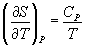→ (10.2)

Therefore,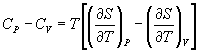→ (10.3)

Considering S as a function of T & V, as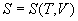differentiating,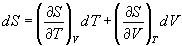→ (10.4)

Dividing Equn.10.4 by dT at constant P,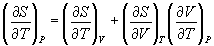i.e.,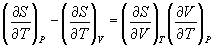→ (10.5)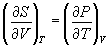Therefore,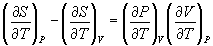→ (10.6)

Cyclic relation rule: (for the function in the variables x, y & z)→ (10.7)

We can write similar relation for the function in the variables P, T & V as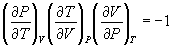→ (10.8)

i.e.,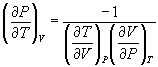→ (10.9)

and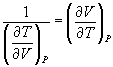→ (10.10)

Therefore,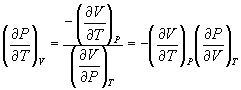→ (10.11)

Substituting for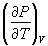from Equn.10.11 in Equn.10.6,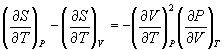→ (10.12)

Substituting from Equn.10.12, in Equn.10.3,→ (10.13)